Processing ......FreeComputerBooks.com Links to Free Computer, Mathematics, Technical Books all over the World

Linear Algebra
🌠 Top Free Mathematics Books - 100% Free or Open Source!
• Title Linear Algebra
• Author(s) Jim Hefferon
• Publisher: Orthogonal Publishing (2020); eBook (Saint Michael's College, Creative Commons Licensed)
• Paperback: 526 pages
• eBook: PDF
• Language: English
• ISBN-10: 1944325115
• ISBN-13: 978-1944325114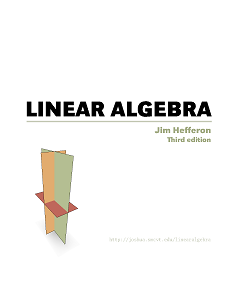Book Description

This textbook covers linear systems and Gauss' method, vector spaces, linear maps and matrices, determinants, and eigenvectors and eigenvalues. Each chapter has three or four discussions of additional topics and applications.

It covers the requisite material and proves all the results, but it does not start by assuming that students are already able at abstract work. Instead, it proceeds with a great deal of motivation, many computational examples, and exercises that range from routine verifications to a few challenges. The goal is, in the context of developing the material of an undergraduate course, to raise each student's level of mathematical maturity.

Reviews, Ratings, and Recommendations: Related Book Categories: Read and Download Links:Similar Books:
•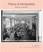Theory of Computation, Making Connections (Jim Hefferon)

The book is geared toward those who thirst for computation theory knowledge. To cater to the demands of a wide range of people, the principles in this book are explained in a way that is easy to understand, digest and apply in the upcoming career.

•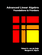Advanced Linear Algebra: Foundations to Frontiers (Robert Geijn)

The focus is on numerical linear algebra, the study of how theory, algorithms, and computer arithmetic interact. These materials keep the learner engaged by intertwining text, videos, exercises, and programming activities in consumable chunks.

•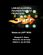Linear Algebra: Foundations to Frontiers (M. Myers, et al.)

This book is an introduction to the basic concepts of linear algebra, along with an introduction to the techniques of formal mathematics. It begins with systems of equations and matrix algebra before moving into the advanced topics.

•Computational Linear Algebra and N-dimensional Geometry

This undergraduate textbook on Linear Algebra and n-Dimensional Geometry, in a self-teaching style, is invaluable for sophomore level undergraduates in mathematics, engineering, business, and the sciences.

•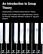An Introduction to Group Theory: Mathematical Music Theory

This is a modern presentation of the fundamental notions of Group Theory is chosen, where the language of commutative diagrams and universal properties, so necessary in Modern Mathematics, in Physics and Computer Science, among other disciplines.

•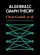Explorations in Algebraic Graph Theory (Chris Godsil, et al.)

This book aims to express properties of graphs in algebraic terms, then to deduce theorems about them. It tackles the applications of linear algebra and matrix theory to the study of graphs; algebraic constructions such as adjacency matrix, using the Sage.

•A First Course in Linear Algebra (Robert A Beezer)

Linear Algebra: Foundations to Frontiers (LAFF) is packed full of challenging, rewarding material that is essential for mathematicians, engineers, scientists, and anyone working with large datasets.

•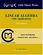Linear Algebra with Applications (W. Keith Nicholson)

The aim of the text is to achieve a balance among computational skills, theory, and applications of linear algebra. It is a relatively advanced introduction to the ideas and techniques of linear algebra targeted for science and engineering students.

•Applied Linear Algebra: Vectors, Matrices, and Least Squares

Combines straightforward explanations with a wealth of practical examples to offer an innovative approach to teaching linear algebra. Requiring no prior knowledge of the subject, it covers the aspects of linear algebra - vectors, matrices, and least squares

•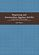Beginning and Intermediate Algebra (Tyler Wallace)

These books use a teacherly writing style and a careful blend of skills development and conceptual questions to meet the unique needs of the developmental math student. It takes advantage of experiences in the classroom and an editing eye to offer one of the most well-rounded series available, written with the developmental learner in mind.

•Linear Algebra: A Course for Physicists and Engineers (Arak Mathai)

This textbook on linear algebra is written to be easy to digest by non-mathematicians. It introduces the concepts of vector spaces and mappings between them without too much theorems and proofs. Various applications of the formal theory are discussed as well.

•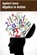Applied Linear Algebra in Action (Vasilios N. Katsikis)

This textbook contains a collection of six high-quality chapters. The techniques are illustrated by a wide sample of applications. This book is devoted to Linear Mathematics by presenting problems in Applied Linear Algebra of general or special interest.

Book Categories
 :All CategoriesTop Free BooksRecent BooksMiscellaneous BooksComputer EngineeringComputer LanguagesComputer ScienceData Science/DatabasesJava and Java EE (J2EE)Linux and UnixMathematicsMicrosoft and .NETMobile ComputingNetworking and CommunicationsSoftware EngineeringSpecial TopicsWeb Programming
Other Categories